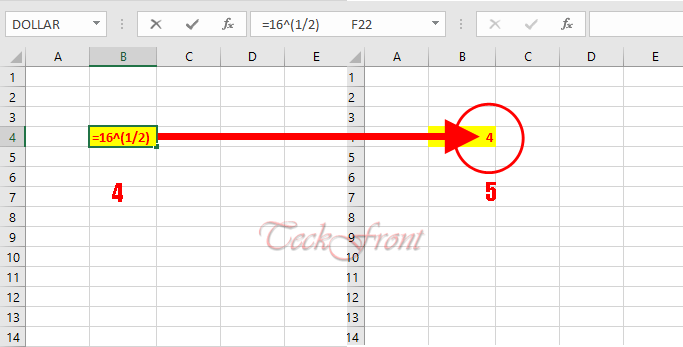# How to Use Exponentiation for Square or Square Root Calculations in Microsoft Excel?

Majority of the students use and apply the functions of Microsoft Excel for a variety of the objectives and studies for the simple and sometimes for advanced and complex calculations. With the passage of the time, great majority of the students are relying upon the Excel formulas for the different functions and operators.

Note: Operators are symbols that are used to point out and indicate the specific mathematical operation which is required by you and which you want the Excel formula to perform the required calculations.

Among many such operators, exponentiation is an important operator which is used to solve many medium levels of calculations. For example, if you are willing to get the square root or cube root or so on, there are many methods in mathematics. But, Excel has suggested a very useful and easy tool to get square root or cube root. This is application of Exponentiation which is symbolized as ‘^’.

To understand this function in a little detail, see our tutorial.

#### Tutorial

##### Square or Power Calculation

1: In the Excel 2016 worksheet, click in any cell.

2: Write the symbol ‘=’ in the cell to start calculation process.

3: Now, write the numeric value for which you want to get square.

4: After the numeric value, write symbol of Exponentiation operator, i.e. ‘^’.

5: After the ‘^’, write 2 – if you are willing to get square raised power of the numeric value to 2. This formula will appear like this: ‘=4^2’.

6: Now, press enter to work with calculation. See, the result in this instance will be: 16.

7: We may also write the same formula like this: ‘=4*4’

8: And its result will still be: ‘16’.9: See the resulting figure will be same in both the calculations. You may substitute any figures in this formula and may raise the power to any other number, depending upon your requirements.

##### Square Root Calculation

If you are willing to work with square roots, the same exponentiation can be of great help for you. Let’s have a look upon the calculations for the simple square root.

2: Write any numeric value after the ‘=’ symbol.

3: After the numeric value, write ‘^’ operator.